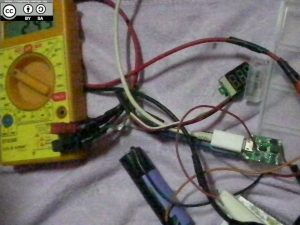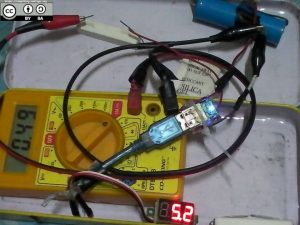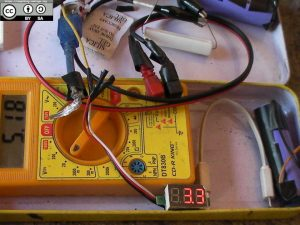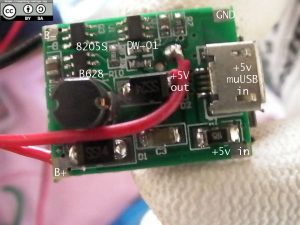Lithium Ion 18650 Tests

18650
18 = length
65 = diameter
0 = cylindrical shape

The batteries that I bought for PHP 20 and the Power Bank does not have any Capacity (mAh) markings on them and I do not have any battery tester or analyzer to determine their capacity.

I only have a 10 ohm 10 Watt, 20 ohm 10 Watt resistors, 5~30V DC voltmeter, Cheap Digital Multimeter, Power Bank Circuit and some wires.

I plan to charge the three batteries full and discharge them using the resistor. The setup is as follows:

The batteries are now fully charged, it took me about 5 hours to completely charge each of them.
I will be using the power bank circuit, connecting the battery and the resistor to the 5V output of the circuit.
Start a stopwatch until the powerbank circuit cuts off the battery, thus preventing it to discharge below 3V.

Disclaimer: These tests may not be 100% accurate, I do not have proper test equipment for these batteries. My computations may also be wrong.

``````
Ambient Temperature Environment: >30 deg Celsius
First test:
1st Battery 18650 PHP 20:
Results:
Charge time: >5 hours
Discharge time: 5 hours
Current throught the resistor (load) from 5V out: 0.38A (not counting the resistance of my multimeter)
Load: 20 ohm 10 Watt resistor
Power = VI = (5.1V)(0.38A) = 1.938 W

Probable capacity of the battery:
Watt-hour = PT = (1.938W)(5hr)
Ampere-hr = (Wh/V) = (9.69Wh/5.1V) = 1.9 Ah = 1900 mAhSecond battery test: (PHP 35 Dead Power Bank)I have tested the `dead` battery and it took me 4 hours to fully charge it and it became hot but did not explode.
Discharging the battery using a load of 10 ohm 10 Watts connected to the 5v output of the Power Bank circuit made it warm, the time it took to fully discharge the cell down to 3.0V is only about 30 minutes.

The Power Bank `dead` battery test:
Battery Voltage: 4.04V
Battery to Power Bank PCB current: N/A
Load (10 ohm 10 W) current to 5v out: 0.46 A
5v output: 5.1V
Power = (5.1V)(0.46A) = 2.346 W
Time to full discharge (3V): ~30 minutes

Probable capacity of the battery:
Wh = (2.346 W)(0.5 hr) = 1.173
Ah = (1.173/5.1) = 0.23 Ah or 230 mA

Third battery test:This is the second PHP 20 18650 battery test, I have also measured the efficiency of the Power Bank circuit (step-up boost).
Results:
Ambient Temperature Environment: >29 deg Celsius
Test 1
Battery Voltage (open circuit): 4.2V
Battery to Power Bank PCB current: 0.41 A
Power = (4.2V)(0.41A) = 1.722 W
Load (20 ohm 10 W) current to 5v out: 0.25 A
5v output: 5.1V
Power = (5.1V)(0.25A) = 1.275 W
Efficiency = Pout/Pin x 100 = (1.275/1.722)(100) = 74 %

Test 2 (Load is ON after 30 minutes)
Battery Voltage: 3.9V
Battery to Power Bank PCB current: 0.43 A
Power = (3.9V)(0.43A) = 1.505 W
Load (20 ohm 10 W) current to 5v out: 0.25 A
5v output: 5.1V
Power = (5.1V)(0.25A) = 1.275 W
Efficiency = Pout/Pin x 100 = (1.275/1.505)(100) = 85 %

Test 3 (Load is ON after 1:57:00)
Battery Voltage: 3.3V
Battery to Power Bank PCB current: 0.45 A
Power = (3.3V)(0.45A) = 1.485 W
Load (20 ohm 10 W) current to 5v out: 0.25 A
5v output: 5.1V
Power = (5.1V)(0.25A) = 1.275 W
Efficiency = Pout/Pin x 100 = (1.275/1.485)(100) = 86 %

Test 4 (Load is ON after 3:44:00)
Battery Voltage: 3.1V
Battery to Power Bank PCB current: 0.50 A
Power = (3.1V)(0.50A) = 1.55 W
Load (20 ohm 10 W) current to 5v out: 0.25 A
5v output: 5.1V
Power = (5.1V)(0.25A) = 1.275 W
Efficiency = Pout/Pin x 100 = (1.275/1.55)(100) = 82 %

Test 5 (Load is ON after 4:47:31)
Battery Voltage: 2.6V
Battery to Power Bank PCB current: 0.70 A
Power = (2.6V)(0.50A) = 1.82 W
Load (20 ohm 10 W) current to 5v out: 0.25 A
5v output: 5.1V
Power = (5.1V)(0.25A) = 1.275 W
Efficiency = Pout/Pin x 100 = (1.275/1.82)(100) = 70 %

Probable capacity of the battery:
Wh = (1.275)(4.78) = 6.09
Ampere-hr = Wh/V = (6.09)/(5.1) = 1.195 Ah or 1195 mAh
``````

Summary:
Load: 20 Ohm and 10 W resistor connected to the 5V output of the Power Bank circuit.
Battery 1 (PHP 20): 1900 mAh
Battery 2 (PHP 20): 1195 mAh

Load: 10 Ohm and 10 W resistor connected to the 5V output of the Power Bank circuit.
Battery 3 (PHP 35 PB): 233 mAh (Dead/Revived Battery)

NOTE:
I used the Power Bank’s PCB to discharge the batteries and I noticed that the PCB does not turn off the load to protect the battery. The load is connected to the +5v out test point that I found, but the COM/GND is connected to the battery minus. The battery minus of course is not protected against overdischarge, so I looked for another test point and it is near the USB sockets that test point (GND) is connected to the protection circuit, so the board shuts down the load to protect the battery.

The protected GND for the load outUPDATE! UPDATE
I have tested the battery 2 using my Battery Capacity Tester and the result was 2310 mAh!